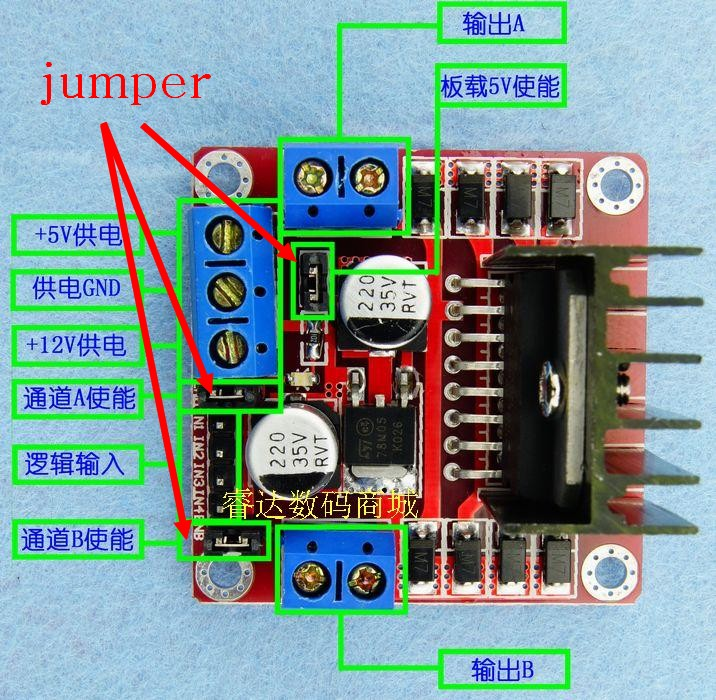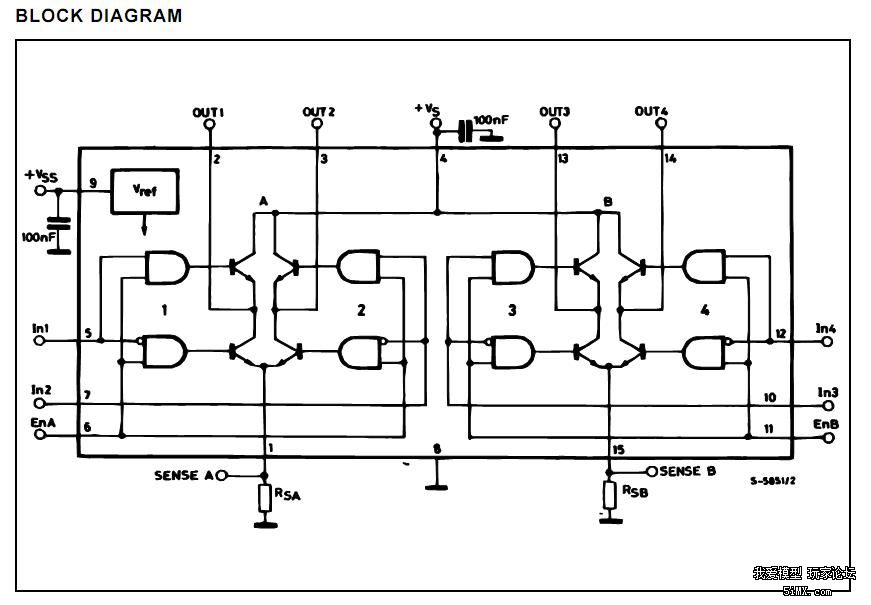# 两相四线步进电机的驱动

1.关于L2982.驱动一个步进电机接线方式：
IN1 IN2 IN3 IN4接开发板的四个管脚，用于给出脉冲；

+5V接开发板的5V,12V接外接电源，GND接外接电源GND，用于给驱动模块以及电机供电，电压不够可能出现电机抖动但是不转动的情况；
ENA ENB 板载5V等分别用跳接帽短接。

3.节拍部分，二相四线可使用4、8节拍

（1）四拍：A 1000，A- 0010,B 0100,B- 0001.
（2）八拍：A 1000，AA- 1010,A- 0010,A-B 0110,B 0100,BB- 0101,B- 0001,B-A 1001.

4.电机固有步距角： 它表示控制系统每发一个步进脉冲信号，电机所转动的角度。

5.步进电机有速度控制与角度控制

6.代码部分：

void MOTOR_Init(void)
{
RCC->APB2ENR|=1<<2;
GPIOA->CRL&=0xffff0000;
GPIOA->CRL|=0x00003333;
}

//第一拍
AIN1_1=1;
AIN1_2=0;
BIN1_1=0;
BIN1_2=0;
delay_us(2500);
//第二拍
AIN1_1=1;
AIN1_2=0;
BIN1_1=1;
BIN1_2=0;
delay_us(2500);
//第三拍
AIN1_1=0;
AIN1_2=0;
BIN1_1=1;
BIN1_2=0;
delay_us(2500);
//第四拍
AIN1_1=0;
AIN1_2=1;
BIN1_1=1;
BIN1_2=0;
delay_us(500);
//第五拍
AIN1_1=0;
AIN1_2=1;
BIN1_1=0;
BIN1_2=0;
delay_us(2500);
//第六拍
AIN1_1=0;
AIN1_2=1;
BIN1_1=0;
BIN1_2=1;
delay_us(2500);
//第七拍
AIN1_1=0;
AIN1_2=0;
BIN1_1=0;
BIN1_2=1;
delay_us(2500);
//第八拍
AIN1_1=1;
AIN1_2=0;
BIN1_1=0;
BIN1_2=1;
delay_us(2500);

TB6612驱动步进电机

GND PWMA
VCC AIN2
AO1 AIN1
AO2 NC
BO2 BIN1
BO1 BIN2
VM PWMB
GND GND

GND接地；
PWMA、PWMB接5V;
AO1、AO2、BO1、BO2分别接步进电机的红绿黄蓝；
AIN1、AIN2、BIN1、BIN2分别接开发板的相应管脚，一一对应；
VM、GND接外接电源；
NC可以悬空。